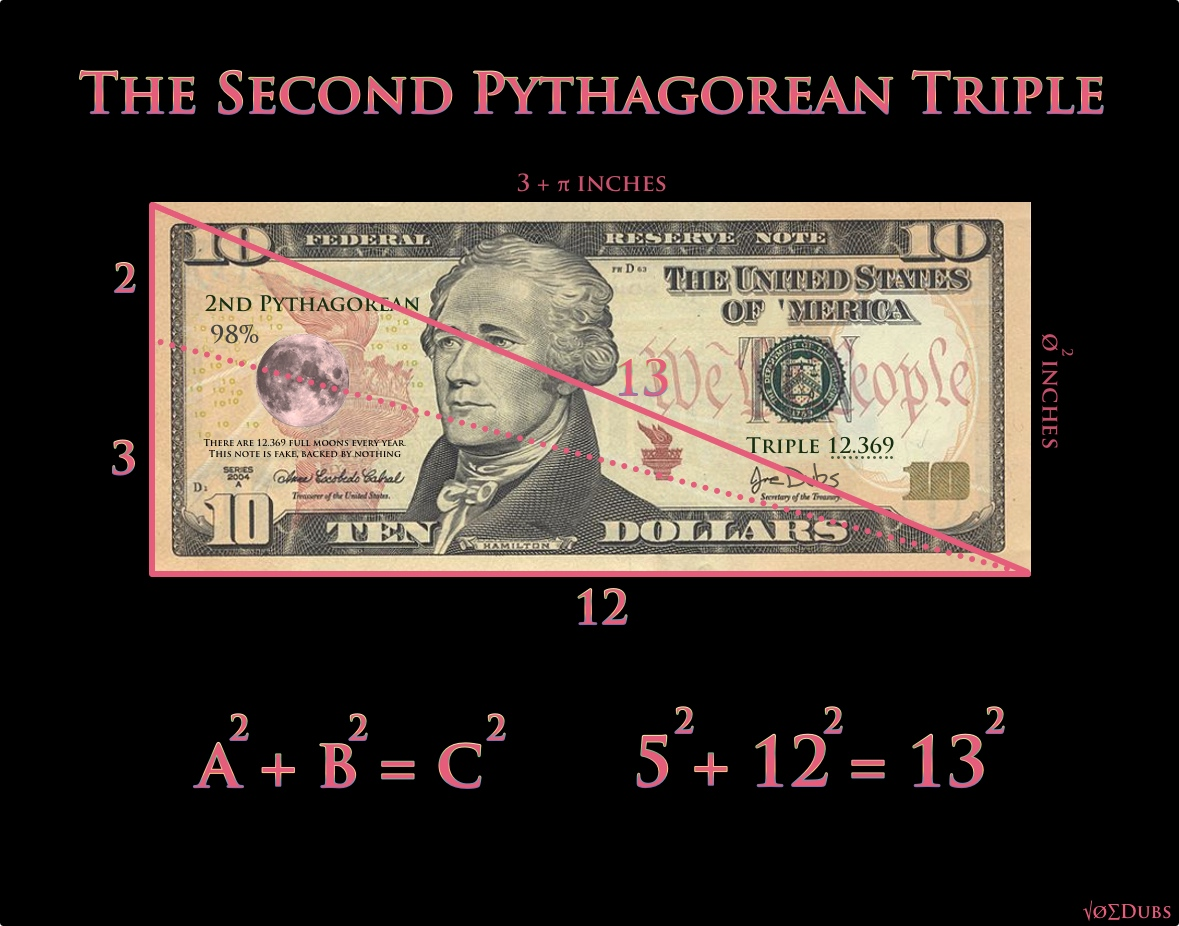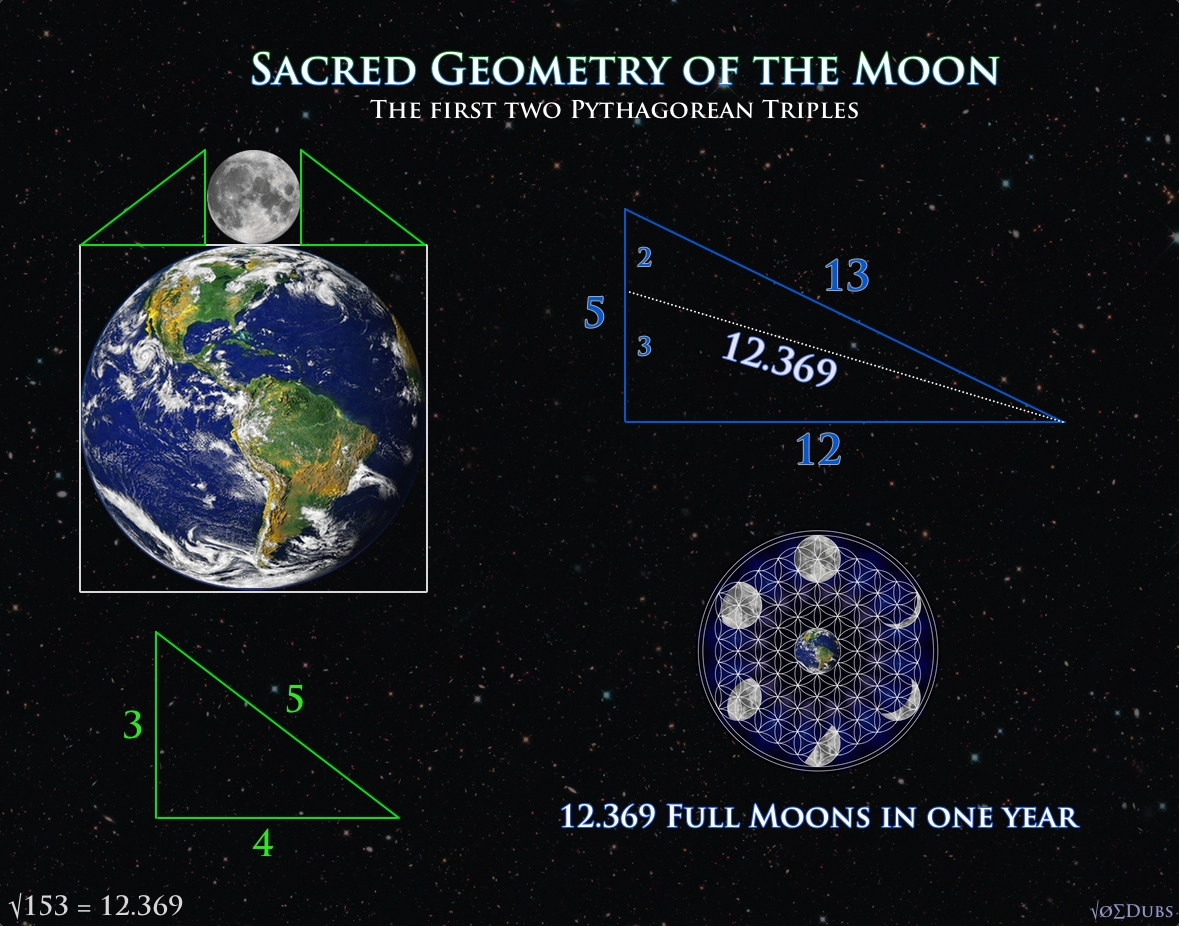## Pythagorean TriplesYou’ve heard of the Pythagorean Theorem. Looking at a triangle, A squared plus B squared equals C squared. But this doesn’t work with all triangles, only specific triangles with specific lengths. The first set of numbers that work for this formula are 3,4 and 5. (3squared=9, 4squared=16, and 5squared equals 25). 9+16=25. Check! This works, so this is the first “Pythagorean Triple”. The second triangle that satisfies the equation is based on lengths of 5,12, and 13. Now if you divide the side of 5, into 2 and 3(The Perfect Fifth in Music Theory), and draw a line as shown(dotted line), it’s length is the amount of full moons in one solar year.(12.369) I wonder if the privately owned Federal Reserve Bank was thinking of the Moon and the second Pythagorean Triple when they came up with the measurements of the US dollar.(The FED…

3

## Sacred Geometry of the MoonThe physical dimensions of Earth and Moon reveal astounding geometries. The sacred geometry of the Moon was understood long ago and the ancients hid the esoteric meaning in the dimensions of their monuments and architecture. The Moon encodes the first two Pythagorean Triples, which are sets of whole numbers that satisfy the equation popularized by Pythagoras that everyone is familiar with. Pythagorean Theorem a^squared + b^squared = c^squared In the book “Taking Measure”, Scott Onstott shows that the point of intersection that defined the amount of full moons in a year (12.369), lies on the perfect fifth (2:3) ratio on the smallest side of the triangle. These numbers also encode esoteric knowledge about the geometric locations of Stonehenge and other sacred sites in England. He continues: “In The Lost Science of Measuring the Earth, John Michell and Robin Heath identified a 5:12:13 Pythagorean triangle…

3